# Python Program to Perform Addition Subtraction Multiplication Division

In this article, we've created some programs in Python, that performs addition, subtraction, multiplication and division of any two numbers entered by user at run-time. Here are the list of programs:

• Simple Add, Subtract, Multiply, and Divide Program
• Add, Subtract, Multiply, and Divide based on User's Choice
• Add, Subtract, Multiply, and Divide using user-defined Function
• Using Class

## Add, Subtract, Multiply, and Divide

To perform addition, subtraction, multiplication and division in Python, you have to ask from user to enter any two numbers. Based on two numbers input, the program find and prints the result for all four operations.

```print("Enter First Number: ")
numOne = int(input())
print("Enter Second Number: ")
numTwo = int(input())
res = numOne+numTwo
res = numOne-numTwo
print("Subtraction Result = ", res)
res = numOne*numTwo
print("Multiplication Result = ", res)
res = numOne/numTwo
print("Division Result = ", res)```

Here is the initial output produced by this Python program: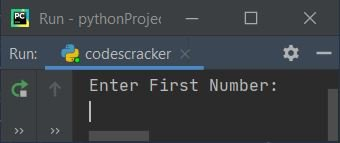Now let's type any two numbers say 6 as first and 3 as second number to perform addition, subtraction, multiplication, and division as shown in the snapshot given below: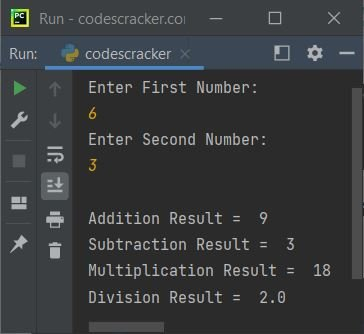Note - You may also like Calculator Program in Python to perform same thing in perfect way.

#### Modified Version of Previous Program

This is the modified version of previous program. The end is used to skip automatic printing of newline through print(). The str() method is used to convert from any type to a string type.

```print("Enter Two Numbers: ", end="")
nOne = int(input())
nTwo = int(input())
print("\n" +str(nOne)+ " + " +str(nTwo)+ " = " +str(nOne+nTwo))
print(str(nOne)+ " - " +str(nTwo)+ " = " +str(nOne-nTwo))
print(str(nOne)+ " * " +str(nTwo)+ " = " +str(nOne*nTwo))
print(str(nOne)+ " / " +str(nTwo)+ " = " +str(nOne/nTwo))```

Here is its sample run with user input, 10 as first and 2 as second number: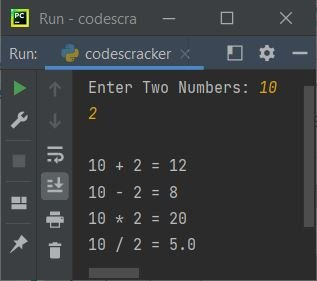### Add, Subtract, Multiply, Divide based on User's Choice

This program performs same operations as done in previous program, but based on user's choice. That is, this program doesn't automatically prints all the four operation's result. Rather it asks from user, and will do the task based on input.

```print("Enter Two Numbers: ", end="")
nOne = int(input())
nTwo = int(input())
print("Enter the Operator (+,-,*,/): ", end="")
ch = input()
if ch=='+':
print("\n" +str(nOne)+ " + " +str(nTwo)+ " = " +str(nOne+nTwo))
elif ch=='-':
print("\n" +str(nOne)+ " - " +str(nTwo)+ " = " +str(nOne-nTwo))
elif ch=='*':
print("\n" +str(nOne)+ " * " +str(nTwo)+ " = " +str(nOne*nTwo))
elif ch=='/':
print("\n" +str(nOne)+ " / " +str(nTwo)+ " = " +str(nOne/nTwo))
else:
print("\nInvalid Operator!")```

Here is its sample run with user input, 10 as first and 20 as second number, / (divide) as operator: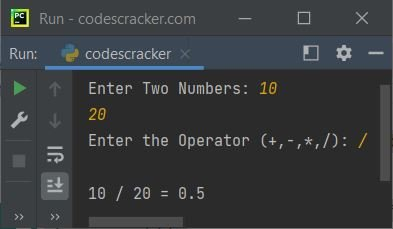Here is another sample run with user input, 5 as first and 0 as second number, then * (multiply) as operator: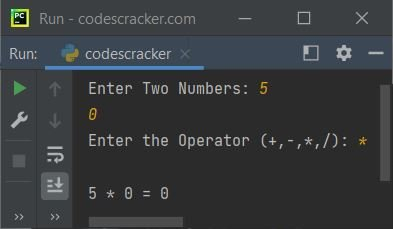## Add, Subtract, Multiply, Divide using Function

This program uses four user-defined functions to perform the thing. That is, this program has four functions namely add(), sub(), mul(), and div(). All functions recevies two argument and returns the corresponding result.

```def add(a, b):
return a+b
def sub(a, b):
return a-b
def mul(a, b):
return a-b
def div(a, b):
return a/b

print("Enter Two Numbers: ", end="")
nOne = int(input())
nTwo = int(input())
print("Enter the Operator (+,-,*,/): ", end="")
ch = input()
if ch=='+':
print("\n" +str(nOne)+ " + " +str(nTwo)+ " = " +str(add(nOne, nTwo)))
elif ch=='-':
print("\n" +str(nOne)+ " - " +str(nTwo)+ " = " +str(sub(nOne, nTwo)))
elif ch=='*':
print("\n" +str(nOne)+ " * " +str(nTwo)+ " = " +str(mul(nOne, nTwo)))
elif ch=='/':
print("\n" +str(nOne)+ " / " +str(nTwo)+ " = " +str(div(nOne, nTwo)))
else:
print("\nInvalid Operator!")```

This program produces the same output as of previous program. Here is its sample run with user input, 5 and 10 as two numbers and - as operator to perform: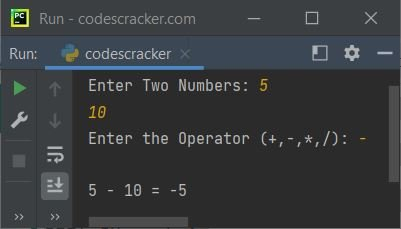## Add, Subtract, Multiply, Divide using Class

This is the last program of this article. It is created using class, an object-oriented feature of Python.

```class CodesCracker:
return a+b
def sub(self, a, b):
return a-b
def mul(self, a, b):
return a-b
def div(self, a, b):
return a/b

print("Enter Two Numbers: ", end="")
nOne = int(input())
nTwo = int(input())
print("Enter the Operator (+,-,*,/): ", end="")
ch = input()

cobj = CodesCracker()

if ch=='+':
print("\n" +str(nOne)+ " + " +str(nTwo)+ " = " +str(cobj.add(nOne, nTwo)))
elif ch=='-':
print("\n" +str(nOne)+ " - " +str(nTwo)+ " = " +str(cobj.sub(nOne, nTwo)))
elif ch=='*':
print("\n" +str(nOne)+ " * " +str(nTwo)+ " = " +str(cobj.mul(nOne, nTwo)))
elif ch=='/':
print("\n" +str(nOne)+ " / " +str(nTwo)+ " = " +str(cobj.div(nOne, nTwo)))
else:
print("\nInvalid Operator!")```

This program also produces similar output as of previous program.

The class CodesCracker is already defined. So using the following statement:

`cobj = CodesCracker()`

All the properties of CodesCracker gets assigned to cobj object. Now we can access member functions of the class CodesCracker using this object through dot (.) operator.

#### Short Version of Previous Program

This is the short version of previous program. In this program, we've applied the condition checking part inside the member function of the class.

```class CodesCracker:
def aOperation(self, a, b, c):
if c=='+':
return a+b
elif c=='-':
return a-b
elif c=='*':
return a*b
elif c=='/':
return a/b
else:
return 'i'

print("Enter Two Numbers: ", end="")
nOne = int(input())
nTwo = int(input())
print("Enter the Operator (+,-,*,/): ", end="")
ch = input()

cobj = CodesCracker()
res = cobj.aOperation(nOne, nTwo, ch)
if res=='i':
print("\nInvalid Operator!")
else:
print("\nResult =", res)```

Here is a sample run with user input 5 as first and -6 as second number, then + as operator to perform: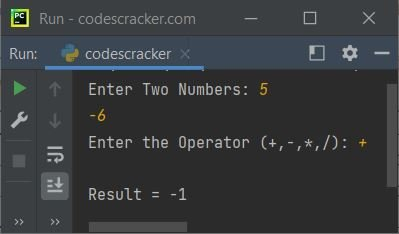#### Same Program in Other Languages

Python Online Test

« Previous Program Next Program »